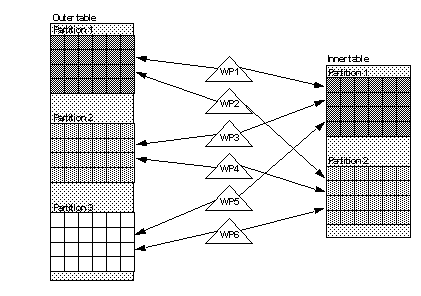# Nested-loop joins

For individual tables in a nested-loop join, the optimizer computes the degree of parallelism using the same rules described in “Optimized degree”. However, the degree of parallelism for the join query as a whole is the product of the worker processes that access individual tables in the join. All worker processes allocated for a join query access all tables in the join. Using the product of worker processes to drive the degree of parallelism for a join ensures that processing is distributed evenly over partitions and that the join returns no duplicate rows.

Figure 25-6 illustrates this rule for two tables in a join where the outer table has three partitions and the inner table has two partitions. If the optimizer determines that partition-based access methods are to be used on each table, then the query requires a total of six worker processes to execute the join. Each of the six worker processes scans one partition of the outer table and one partition of the inner table to process the join condition.

Figure 25-6: Worker process usage for a nested-loop joinIn Figure 25-6, if the optimizer chose to scan the inner table using a serial access method, only three worker processes would be required to execute the join. In this situation, each worker process would scan one partition of the outer table, and all worker processes would scan the inner table to find matching rows.

Therefore, for any two tables in a query with scan degrees of m and n respectively, the potential degrees of parallelism for a nested-loop join between the two tables are:

• 1, if the optimizer accesses both tables serially

• m*1, if the optimizer accesses the first table using a parallel access method (with m worker processes), and the second table serially

• n*1, if the optimizer accesses the second table using a parallel access method (with n worker processes) and the first table serially

• m*n, if the optimizer accesses both tables using parallel access methods